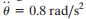### Create an Account

Home / Questions / The 0.5-lb ball is guided along the vertical circular path r = 2rc cos θ using the arm OA...

# The 0.5-lb ball is guided along the vertical circular path r = 2rc cos θ using the arm OA If the arm has an angular velocity θ = 0.4 rad/s and an angular acceleration

The 0.5-lb ball is guided along the vertical circular path r = 2rc cos θ  using the arm OA. If the arm has an angular velocity θ = 0.4 rad/s and an angular accelerationat the instant θ = 30° , determine the force of the arm on the ball. Neglect friction and the size of the ball set rc = 0.4 ft

Jun 03 2020 View more View LessSubscribe To Get Solution